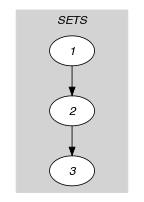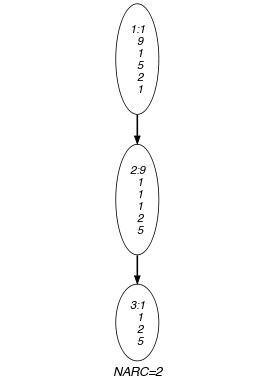## 5.211. k_used_by

Origin
Constraint

$𝚔_\mathrm{𝚞𝚜𝚎𝚍}_\mathrm{𝚋𝚢}\left(\mathrm{𝚂𝙴𝚃𝚂}\right)$

Type
 $\mathrm{𝚅𝙰𝚁𝙸𝙰𝙱𝙻𝙴𝚂}$ $\mathrm{𝚌𝚘𝚕𝚕𝚎𝚌𝚝𝚒𝚘𝚗}\left(\mathrm{𝚟𝚊𝚛}-\mathrm{𝚍𝚟𝚊𝚛}\right)$
Argument
 $\mathrm{𝚂𝙴𝚃𝚂}$ $\mathrm{𝚌𝚘𝚕𝚕𝚎𝚌𝚝𝚒𝚘𝚗}\left(\mathrm{𝚜𝚎𝚝}-\mathrm{𝚅𝙰𝚁𝙸𝙰𝙱𝙻𝙴𝚂}\right)$
Restrictions
 $\mathrm{𝚛𝚎𝚚𝚞𝚒𝚛𝚎𝚍}$$\left(\mathrm{𝚅𝙰𝚁𝙸𝙰𝙱𝙻𝙴𝚂},\mathrm{𝚟𝚊𝚛}\right)$ $|\mathrm{𝚅𝙰𝚁𝙸𝙰𝙱𝙻𝙴𝚂}|\ge 1$ $\mathrm{𝚛𝚎𝚚𝚞𝚒𝚛𝚎𝚍}$$\left(\mathrm{𝚂𝙴𝚃𝚂},\mathrm{𝚜𝚎𝚝}\right)$ $|\mathrm{𝚂𝙴𝚃𝚂}|>1$ $\mathrm{𝚗𝚘𝚗}_\mathrm{𝚒𝚗𝚌𝚛𝚎𝚊𝚜𝚒𝚗𝚐}_\mathrm{𝚜𝚒𝚣𝚎}$$\left(\mathrm{𝚂𝙴𝚃𝚂},\mathrm{𝚜𝚎𝚝}\right)$
Purpose

Given $|\mathrm{𝚂𝙴𝚃𝚂}|$ sets of domain variables, the $𝚔_\mathrm{𝚞𝚜𝚎𝚍}_\mathrm{𝚋𝚢}$ constraint forces a $\mathrm{𝚞𝚜𝚎𝚍}_\mathrm{𝚋𝚢}$ constraint between each pair of consecutive sets.

Example
$\left(\begin{array}{c}〈\begin{array}{c}\mathrm{𝚜𝚎𝚝}-〈1,9,1,5,2,1〉,\hfill \\ \mathrm{𝚜𝚎𝚝}-〈9,1,1,1,2,5〉,\hfill \\ \mathrm{𝚜𝚎𝚝}-〈1,1,2,5〉\hfill \end{array}〉\hfill \end{array}\right)$

The $𝚔_\mathrm{𝚞𝚜𝚎𝚍}_\mathrm{𝚋𝚢}$ constraint holds since:

• The multiset of values $\left\{\left\{1,1,1,2,5,9\right\}\right\}$ associated with the second collection of variables is included into the multiset $\left\{\left\{1,1,1,2,5,9\right\}\right\}$ associated with the first collection of variables.

• The multiset of values $\left\{\left\{1,1,2,5\right\}\right\}$ associated with the third collection of variables is included into the multiset $\left\{\left\{1,1,1,2,5,9\right\}\right\}$ associated with the second collection of variables.

Typical
$|\mathrm{𝚅𝙰𝚁𝙸𝙰𝙱𝙻𝙴𝚂}|>1$
Symmetries
• Items of $\mathrm{𝚂𝙴𝚃𝚂}$ are permutable.

• Items of $\mathrm{𝚂𝙴𝚃𝚂}.\mathrm{𝚜𝚎𝚝}$ are permutable.

• All occurrences of two distinct values of $\mathrm{𝚂𝙴𝚃𝚂}.\mathrm{𝚜𝚎𝚝}.\mathrm{𝚟𝚊𝚛}$ can be swapped; all occurrences of a value of $\mathrm{𝚂𝙴𝚃𝚂}.\mathrm{𝚜𝚎𝚝}.\mathrm{𝚟𝚊𝚛}$ can be renamed to any unused value.

Arg. properties

Contractible wrt. $\mathrm{𝚂𝙴𝚃𝚂}$.

Remark

Similarly to the $𝚔_\mathrm{𝚜𝚊𝚖𝚎}$ constraint [ElbassioniKatrielKutzMahajan05], finding out whether the $𝚔_\mathrm{𝚞𝚜𝚎𝚍}_\mathrm{𝚋𝚢}$ constraint has a solution or not is NP-hard when we have more than one $\mathrm{𝚞𝚜𝚎𝚍}_\mathrm{𝚋𝚢}$ constraint.

Keywords
Arc input(s)

$\mathrm{𝚂𝙴𝚃𝚂}$

Arc generator
$\mathrm{𝑃𝐴𝑇𝐻}$$↦\mathrm{𝚌𝚘𝚕𝚕𝚎𝚌𝚝𝚒𝚘𝚗}\left(\mathrm{𝚜𝚎𝚝}\mathtt{1},\mathrm{𝚜𝚎𝚝}\mathtt{2}\right)$

Arc arity
Arc constraint(s)
$\mathrm{𝚞𝚜𝚎𝚍}_\mathrm{𝚋𝚢}$$\left(\mathrm{𝚜𝚎𝚝}\mathtt{1}.\mathrm{𝚜𝚎𝚝},\mathrm{𝚜𝚎𝚝}\mathtt{2}.\mathrm{𝚜𝚎𝚝}\right)$
Graph property(ies)
$\mathrm{𝐍𝐀𝐑𝐂}$$=|\mathrm{𝚂𝙴𝚃𝚂}|-1$

Graph model

Parts (A) and (B) of Figure 5.211.1 respectively show the initial and final graph associated with the Example slot. To each vertex corresponds a collection of variables, while to each arc corresponds a $\mathrm{𝚞𝚜𝚎𝚍}_\mathrm{𝚋𝚢}$ constraint.

##### Figure 5.211.1. Initial and final graph of the $𝚔_\mathrm{𝚞𝚜𝚎𝚍}_\mathrm{𝚋𝚢}$ constraint(a) (b)